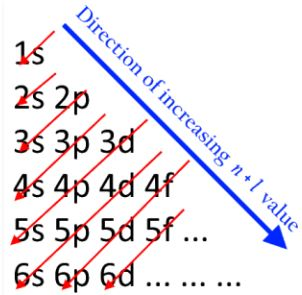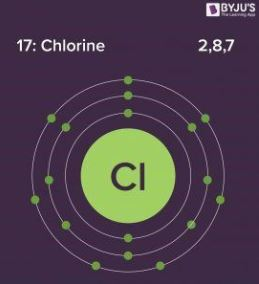Electronic Configuration

The electronic configuration of an element describes how electrons are distributed in its atomic orbitals. Electronic configurations of elements describe the types of chemical bonds formed by the element. Elements with similar electron configurations generally exhibit similar properties.

The valency of an element is often determined via its electronic configuration and with the help of a simplified form of the molecular orbital theory. Another application of this concept is its role in the interpretation of atomic spectra.

Writing Electronic Configurations

Shells

The maximum number of electrons that can be accommodated in a shell is based on the principal quantum number (n). It is represented by the formula 2n2, where ‘n’ is the shell number. The shells, values of n, and the total number of electrons that can be accommodated are tabulated below.

 Shell and ‘n’ value Max. Electrons in the Electronic Configuration K shell, n=1 2*12 = 2 L shell, n=2 2*22 = 8 M shell, n=3 2*32 = 18 N shell, n=4 2*42 = 32

Subshells

• The subshells into which electrons are distributed are based on the azimuthal quantum number (denoted by ‘l’).
• This quantum number is dependant on the value of the principal quantum number, n. Therefore, when n has a value of 4, four different subshells are possible.
• When n=4. the subshells correspond to l=0, l=1, l=2, and l=3 and are named the s, p, d, and f subshells respectively.
• The maximum number of electrons that can be accommodated by a subshell is given by the formula 2*(2l + 1).
• Therefore, the s, p, d, and f subshells can accommodate a maximum of 2, 6, 10, and 14 electrons respectively.

All the possible subshells for values of n up to 4 are tabulated below.

 Principle Quantum Number Value Value of Azimuthal Quantum Number Resulting Subshell in the Electronic Configuration n=1 l=0 1s n=2 l=0 2s l=1 2p n=3 l=0 3s l=1 3p l=2 3d n=4 l=0 4s l=1 4p l=2 4d l=3 4f

Thus, it can be understood that the 1p, 2d, and 3f orbitals do not exist because the value of the azimuthal quantum number is always less than that of the principal quantum number.

Notation

• The electron configuration of an atom is written with the help of subshell labels.
• These labels contain the shell number (given by the principal quantum number), the subshell name (given by the azimuthal quantum number), and the total number of electrons in the subshell in superscript.
• For example, if two electrons are filled in the ‘s’ subshell of the first shell, the resulting notation is ‘1s2’.
• With the help of these subshell labels, the electronic configuration of magnesium (atomic number 12) can be written as 1s2 2s2 2p6 3s2.

Filling of Atomic Orbitals

Aufbau Principle

• This principle is named after the German word ‘Aufbeen’ which means ‘build up’.
• The Aufbau principle dictates that electrons will occupy the orbitals having lower energies before occupying higher energy orbitals.
• The energy of an orbital is calculated by the sum of the principal and the azimuthal quantum numbers.
• According to this principle, electrons are filled in the following order: 1s, 2s, 2p, 3s, 3p, 4s, 3d, 4p, 5s, 4d, 5p, 6s, 4f, 5d, 6p, 7s, 5f, 6d, 7p…

The order in which electrons are filled in atomic orbitals as per the Aufbau principle is illustrated below.It is important to note that there exist many exceptions to the Aufbau principle such as chromium and copper. These exceptions can sometimes be explained by the stability provided by half-filled or completely filled subshells.

Pauli Exclusion Principle

• The Pauli exclusion principle states that a maximum of two electrons, each having opposite spins, can fit in an orbital.
• This principle can also be stated as “no two electrons in the same atom have the same values for all four quantum numbers”.
• Therefore, if the principal, azimuthal, and magnetic numbers are the same for two electrons, they must have opposite spins.

Hund’s Rule

• This rule describes the order in which electrons are filled in all the orbitals belonging to a subshell.
• It states that every orbital in a given subshell are singly occupied by electrons before a second electron is filled in an orbital.
• In order to maximize the total spin, the electrons in the orbitals that only contain one electron all have the same spin (or the same values of the spin quantum number).

Examples

The electronic configurations of a few elements are provided with illustrations in this subsection.

Electronic Configuration of Hydrogen

The atomic number of hydrogen is 1. Therefore, a hydrogen atom contains 1 electron, which will be placed in the s subshell of the first shell/orbit. The electronic configuration of hydrogen is 1s1, as illustrated below.Electronic Configuration of Oxygen

The atomic number of oxygen is 8, implying that an oxygen atom holds 8 electrons. Its electrons are filled in the following order:

K shell – 2 electrons

L shell – 6 electrons

Therefore, the electronic configuration of oxygen is 1s2 2s2 2p4, as shown in the illustration provided below.Chlorine Electronic Configuration

Chlorine has an atomic number of 17. Therefore, its 17 electrons are distributed in the following manner:

K shell – 2 electrons

L shell – 8 electrons

M shell – 7 electrons

The electron configuration of chlorine is illustrated below. It can be written as 1s22s22p63s23p5 or as [Ne]3s23p5